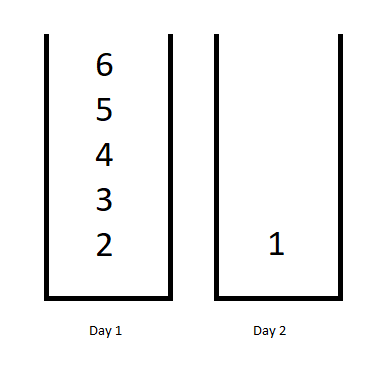# 1335. Minimum Difficulty of a Job Schedule

Hard
You want to schedule a list of jobs in `d` days. Jobs are dependent (i.e To work on the `ith` job, you have to finish all the jobs `j` where `0 <= j < i`).
You have to finish at least one task every day. The difficulty of a job schedule is the sum of difficulties of each day of the `d` days. The difficulty of a day is the maximum difficulty of a job done on that day.
You are given an integer array `jobDifficulty` and an integer `d`. The difficulty of the `ith` job is `jobDifficulty[i]`.
Return the minimum difficulty of a job schedule. If you cannot find a schedule for the jobs return `-1`.
Example 1:Input: jobDifficulty = [6,5,4,3,2,1], d = 2
Output:
7
Explanation:
First day you can finish the first 5 jobs, total difficulty = 6.
Second day you can finish the last job, total difficulty = 1.
The difficulty of the schedule = 6 + 1 = 7
Example 2:
Input: jobDifficulty = [9,9,9], d = 4
Output:
-1
Explanation:
If you finish a job per day you will still have a free day. you cannot find a schedule for the given jobs.
Example 3:
Input: jobDifficulty = [1,1,1], d = 3
Output:
3
Explanation:
The schedule is one job per day. total difficulty will be 3.
Constraints:
• `1 <= jobDifficulty.length <= 300`
• `0 <= jobDifficulty[i] <= 1000`
• `1 <= d <= 10`

### 解題

func minDifficulty(jobDifficulty []int, d int) int {
n:= len(jobDifficulty)
if (d > n) { return -1 }
var dp = make([][]int, d+1)
for i := range dp {
dp[i] = make([]int, n+1)
for j:= 0; j<n+1; j++ {
dp[i][j] = math.MaxInt
}
}
dp = 0
for day:= 1; day<=d; day++ {